Courses

# Turbulent Flow Notes | EduRev

## Civil Engineering (CE) : Turbulent Flow Notes | EduRev

The document Turbulent Flow Notes | EduRev is a part of the Civil Engineering (CE) Course Fluid Mechanics.
All you need of Civil Engineering (CE) at this link: Civil Engineering (CE)

Turbulent Flow

Turbulent flow is a flow regime characterized by the following points as given below.
Shear stress in the turbulent flow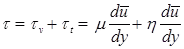where, τv and τt = shear stress due to viscosity and turbulence.
η = eddy viscosity coefficient.

Turbulent shear stress by Reynolds

τ = ρu'v'
u' and v’ fluctuating component of velocity.

Shear stress in turbulent flow by Prandtl

τ = ρl2(du/dy)2
where, l = Mixing length
The velocity distribution in the turbulent flow for pipes is given by the expression.

u = umax + 2.5u*logey/R
Umax = centre velocity
where, y = Distance from the pipe wall, R = radius of the pipe
u* = Shear velocity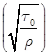Velocity defect is the difference between the maximum velocity (umax) and local velocity (u) at any point is given by
umax -u = 5.75u*log10R/y

Karman-Prandtl velocity distribution equation

Hydrodynamically pipe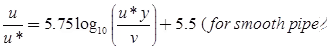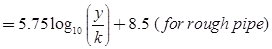where, u = velocity at any point in the turbulent flow
u* = shear velocity =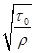v = Kinematic viscosity of fluid
y = Distance from the pipe wall
k = Roughness factor
Velocity distribution in terms of average velocity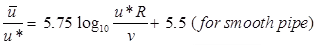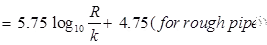Common Mean Velocity Distribution Equation

[(u - u¯) / u*] = 5.75 log10 (y/R) + 3.75
(This is valid for both rough and smooth pipes, that's why it is referred to as Common Mean Velocity Distribution Equation)
Coefficient of friction f = 16/R (for laminar flow)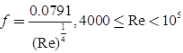(for smooth pipe)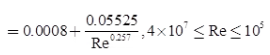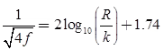(for rough pipe)

Offer running on EduRev: Apply code STAYHOME200 to get INR 200 off on our premium plan EduRev Infinity!

## Fluid Mechanics

43 videos|70 docs|72 tests

,

,

,

,

,

,

,

,

,

,

,

,

,

,

,

,

,

,

,

,

,

;